zizou
★

Plzeň, Czech Republic,
2017-02-05 02:03
(1451 d 03:30 ago)

Posting: # 17014
Views: 22,866

## Denmark Curiosa (1 in 90% CI in 0.8-1.25) [Power / Sample Size]

Hi everybody and nobody.

It was mentioned here several times that Danish Medicines Agency has one more requirement for BE:

"In the opinion of the Danish Medicines Agency, the 90 % confidence intervals for the ratio of the test and reference products should include 100 %, regardless that the acceptance limits are within 80.00-125.00 % or a narrower interval. Deviations are usually only accepted if it can be adequately proved that the deviation has no clinically relevant impact on the efficacy and safety of the medicinal product." source (bolded by me)

Btw. FDA document (Guidance for Industry: Bioavailability and Bioequivalence Studies for Orally Administered Drug Products - General Considerations) states in section II. BACKGROUND:

C. Bioequivalence
Bioequivalence is defined in § 320.1 as:
the absence of a significant difference in the rate and extent to which the active ingredient or active moiety in pharmaceutical equivalents or pharmaceutical alternatives becomes available at the site of drug action when administered at the same molar dose under similar conditions in an appropriately designed study.

When value 1 is outside of 90% CI of GMR then there is a significant difference (maybe except for some border cases). - End of fun, of course this statistically significant difference is not relevant for FDA according to other statements requiring the confidence interval in the BE limits.
On the other hand, if some new regulatory reader is suprised with the significant difference between IMPs (acc. to ANOVA p value) and ask for justification. He would be probably shocked by the answer: "It is not possible to plan the BE study with assumention of GMR=0.95, CV=x%, 90% CI in 0.8-1.25 and not significant treatment effect for power at least 80%."

Maybe, Denmark has the requirement as a way to keep "Forced BE" away.
(Sounds good, until the problems appear and it can filter not only "Forced BE" as everyone knows.)
Nevertheless the EMA's statement "The number of subjects to be included in the study should be based on an appropriate sample size calculation." should be sufficient (to keep "Forced BE" away), if controlled correctly.
(Many of regulatory authorities think that sample size is issue of ethical committees. Many of ethical committees are not able to assess the sample size estimation.)

Back to the future:
Should be the requirement of the inclusion of 100% in 90% CI included in the sample size estimation? (I would bet it has never been used.) ... It's quite problematic task.
(So this post is only for interest to know. - I can not imagine the actual use after I made some power analysis.)

One issue is that with increasing sample size we will get 100% out of 90% CI (Helmut the Hero showed it graphically here).
Another issue is that test and reference products can differ (eg. the assayed content can differ up to 5%, even if the difference is zero (according to CoAs), there is some analytical accuracy, etc.), so it is usual to use null ratio 0.95 for sample size estimation (and possible worse ratio to 0.9 as option - discussed here).
(Null ratio 0.90 would be unlucky coin flipping for Denmark.)

I performed some simulations for power (with added condition of 1 in 90% CI to the condition of 90% CI in 0.8-1.25 (in counting step)).
So I can share my figures (more accurately levelplots) for different null ratio (expected GMR used for power estimation via simulations):

                GMR = 0.95                                GMR = 0.96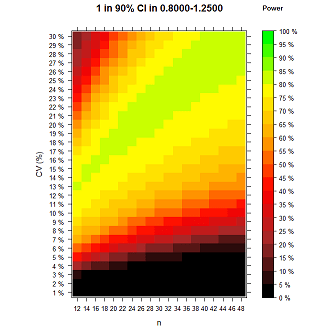For GMR = 0.95 (left side) the maximum simulated power is 0.7809. (For n 12-80 and CV 1-50 maximum simulated power for GMR=0.95 is 0.7888.)
Nice to know that with all these requirements+assumptions the power is below 80%. (Or at least seems to be acc. to simulations.)

                GMR = 0.97                                GMR = 0.98GMR = 0.99                                GMR = 1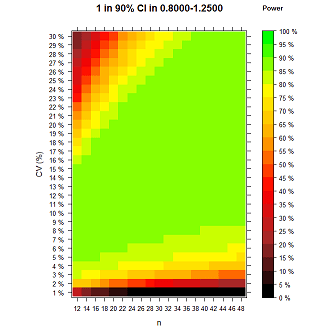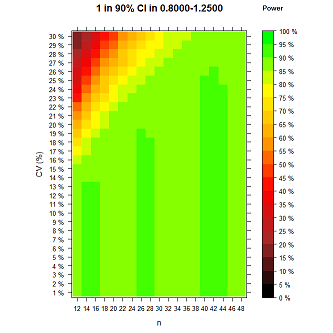For GMR = 1 (right side) the maximum simulated power is 0.9016.
Green columns are little defect due to I decided to move set.seed function into loops (so same seed was used for each combination of n and intra-subject CV, which looks better for smooth color transitions. And I decided to use only 20 colors for easy to see which value is over 80% and which over 90%. So I prepare two additional figures.)
The darker green columns are saying that values are in 0.9-0.95, but the values are close to 0.9, and similar issue in the lighter green category 0.85-0.9 - almost in whole green region all values are really close to 0.9.)
And columns appeared because n+2 makes little bit more change than CV+1 (with the same seed used).

             GMR = 1 (additional 1)                    GMR = 1 (additional 2)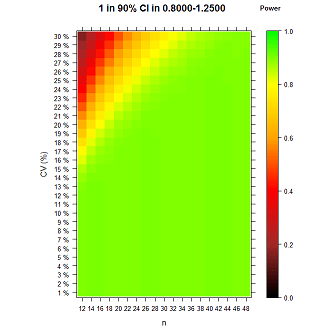GMR = 1 - additional 1 (left side): Function set.seed is still in loops so there is trend in coloring. (Green color is close to 0.9 in the scale.)
GMR = 1 - additional 2 (right side): Function set.seed moved before loops so values are over 90% randomly - it's the same as using of different seed for each combo of n and CV. (Actually there are many of values around 90% power - simulated values are sometimes little bit over 90% - because it is not exact method of course.)
For GMR = 1 (right side, with set.seed before loops) the maximum simulated power is 0.9073.

Null GMR 1, max power 0.90 with alpha 0.05. The simulations confirm what I never thought about but what everyone can expect.
Really scary when sponsor wants to plan the BE study with power 90%. You must expect the GMR value equal to 1 and anyway power 90% is just a theoretical maximum, in practice the power is always lower than 90%. Moreover I ignore that this should be valid for two PK metrics (AUC and Cmax).

GMR = 0.9For GMR = 0.9 the maximum simulated power is 0.4757.

Little bit going to extreme n and CV (presented twice - 1) on the left to easy see categories; 2) on the right as presentable;)
             GMR = 0.95 (20 colors)                    GMR = 0.95 (200 colors)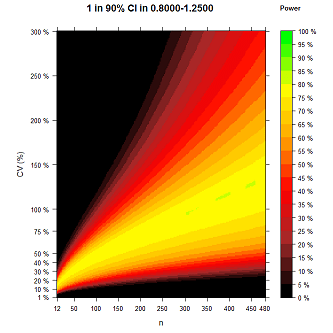For GMR = 0.95 the maximum simulated power is 0.8012 (n=12-480 and CV=1-300%). It can be over 0.8 only due to lucky number as a seed used in simulations, etc..

With comparison to EMA: (I used the same simulations as for Denmark requirement "1 in 90% CI in 0.8-1.25", although exact method exist for standard "90% CI in 0.8-1.25").

GMR = 1:Green area: For Denmark almost 90% power, standardly almost 100% power. It's a big difference!

GMR = 0.95: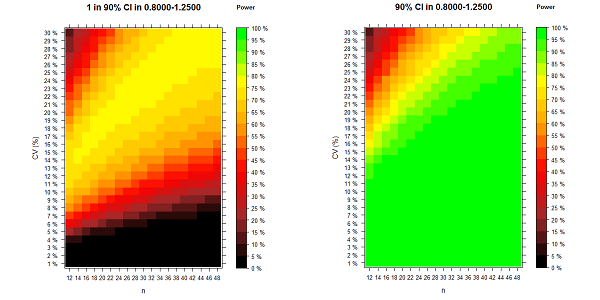No green area for Denmark at all!

GMR = 0.9:No comment!

Coded (with the use of function provided by Hero d_labes here) as:
 power.sim2x2 <- function(CV, GMR, n, nsims=1E4, alpha=0.05, lBEL=0.8, uBEL=1.25, details=FALSE) {   ptm <- proc.time()   # n is total if given as simple number   # to be correct n must then be even!   if (length(n)==1) {     nsum <- n     fact <- 2/n   }   if (length(n)==2) {     nsum <- sum(n)     fact <- 0.5*sum(1/n)   }   mse    <- log(1.0 + CV^2)   df     <- nsum-2   tval   <- qt(1-alpha,df)   # Attention! With nsims=1E8 memory of my machine (4 GB) is too low   # Thus work in chunks of 1E6 if nsims>1E6.   chunks <- 1   ns     <- nsims   if (nsims>1e6) {     chunks <- trunc(nsims/1E6)     ns     <- 1E6     if (chunks*1e6!=nsims){       nsims <- chunks*1e6       warning("nsims truncated to", nsims)     }   }   BEcount <- 0   sdm     <- sqrt(fact*mse)   mlog    <- log(GMR)   for (i in 1:chunks)   {     # simulate sample mean via its normal distribution     means  <- rnorm(ns, mean=mlog, sd=sdm)     # simulate sample mse via chi-square distribution of df*mses/mse     mses   <- mse*rchisq(ns,df)/df     hw     <- tval*sqrt(fact*mses)     lCL <- means - hw     uCL <- means + hw     # point  <- exp(means)     lCL    <- exp(lCL)     uCL    <- exp(uCL)     #BE     <- (lBEL<=lCL & uCL<=uBEL) # standard check if 90% CI of GMR is in 0.8-1.25 (without rounding CI limits)     BE <- lBEL<=lCL & uCL<=uBEL & lCL<=1 & 1<=uCL # change for Denmark     BEcount <- BEcount + sum(BE)   }   if (details) {     cat(nsims,"sims. Time elapsed (sec):\n")     print(proc.time()-ptm)   }   BEcount/nsims } n=seq(12,48,2) CV=seq(1,30,1) power_sim=matrix(0,nrow=length(CV),ncol=length(n)) for (i in 1:length(CV)){  for (j in 1:length(n)){   set.seed(12345) # if set.seed is done before the "for loop", it generate differ set for each n and CV combination   power_sim[i,j]=power.sim2x2(CV=CV[ i ]/100,GMR=0.95,n=n[j],nsims=1E4, alpha=0.05, lBEL=0.8, uBEL=1.25) # I have it without rounding 90% confinfidence limits and with added condition of 1 in 90% CI  } } max(power_sim)  # I know, someone can do it better (eg. in 3D like this kind of figure).  # But not me. x) library(lattice) x <- n y <- CV z <- t(power_sim) rownames(z) <- x colnames(z) <- paste(as.character(y),"%") ck=list(at=seq(0,1,0.05),labels=c("0 %","5 %","10 %","15 %","20 %","25 %","30 %","35 %","40 %","45 %","50 %","55 %","60 %","65 %","70 %","75 %","80 %","85 %","90 %","95 %","100 %")) levelplot(z, at=seq(0,1,length=21), colorkey=ck, col.regions=colorRampPalette(c("black","brown","red","orange","yellow","green")), xlab="n", ylab="CV (%)", main=list(c("                                                 1 in 90% CI in 0.8000-1.2500","                                       Power"),cex=c(1.2,0.8)))  # or below is better looking #levelplot(z, col.regions=colorRampPalette(c("black","brown","red","orange","yellow","green")), at=seq(0,1,length=201), xlab="n", ylab="CV (%)", main=list(c("                                                 1 in 90% CI in 0.8000-1.2500","                                       Power"),cex=c(1.2,0.8)))  # -----  # for levelplot of extreme n and CV: n=seq(12,480,2); CV=seq(1,300,1); #x_ticks=c(1,20,45,70,95,120,145,170,195,220,235) #y_ticks=c(1,10,20,30,40,50,75,100,150,200,250,300) #levelplot(z, at=seq(0,1,length=21), colorkey=ck, col.regions=colorRampPalette(c("black","brown","red","orange","yellow","green")), scales=list(x=list(at=x_ticks,labels=n[x_ticks]),y=list(at=y_ticks,labels=sprintf("%s %%",y_ticks))), xlab="n", ylab="CV (%)", main=list(c("                                                 1 in 90% CI in 0.8000-1.2500","                                               Power"),cex=c(1.2,0.8)))  # or below is better looking #levelplot(z, col.regions=colorRampPalette(c("black","brown","red","orange","yellow","green")), at=seq(0,1,length=201), scales=list(x=list(at=x_ticks,labels=n[x_ticks]),y=list(at=y_ticks,labels=sprintf("%s %%",y_ticks))), xlab="n", ylab="CV (%)", main=list(c("                                                 1 in 90% CI in 0.8000-1.2500","                                               Power"),cex=c(1.2,0.8))) 
In this case, it's more than true to say:

Power. That which statisticians are always calculating
but never have. Stephen Senn internal source

Wingardium Leviosa,
zizou
Helmut
★★★Vienna, Austria,
2017-02-05 19:16
(1450 d 10:17 ago)

@ zizou
Posting: # 17015
Views: 21,427

## Kudos!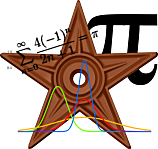Hi zizou,

what a fantastic post deserving a barnstar! First comments (others later, time allowing).

» FDA […] states in section II. BACKGROUND:

C. Bioequivalence
» Bioequivalence is defined in § 320.1 as:
» the absence of a significant difference in the rate and extent to which the active ingredient or active moiety in pharmaceutical equivalents or pharmaceutical alternatives becomes available at the site of drug action when administered at the same molar dose under similar conditions in an appropriately designed study.

» When value 1 is outside of 90% CI of GMR then there is a significant difference (maybe except for some border cases). - End of fun, of course this statistically significant difference is not relevant for FDA according to other statements requiring the confidence interval in the BE limits.

The guidance quotes the definition given in the 21CFR320.1. Hence, it refers to a law. Difficult to change (if you are not Mr Trump). I guess it goes back to the 1980s where no statistics were used at all (the crazy 75/75-rule). Therefore, I also guess that significant is not meant in the statistical sense. Merriam-Webster tells me:
1. having meaning; especially: suggestive <a significant glance>
1. having or likely to have influence or effect: important <a significant piece of legislation>; also: of a noticeably or measurably large amount <a significant number of layoffs> <producing significant profits>
2. probably caused by something other than mere chance <statistically significant correlation between vitamin deficiency and disease>
Does the FDA’s definition say “statistically significant”? Nope. The definition means 2.a. and not 2.b.

» Nevertheless the EMA's statement "The number of subjects to be included in the study should be based on an appropriate sample size calculation." should be sufficient (to keep "Forced BE" away), if controlled correctly.

This “appropriate” drives me nuts. Borrowed from ICH-E9. All previous versions of the BE-GL (or NfG) were better in this respect, IMHO.

» (Many of regulatory authorities think that sample size is issue of ethical committees. Many of ethical committees are not able to assess the sample size estimation.)

Yes to both.

» Should be the requirement of the inclusion of 100% in 90% CI included in the sample size estimation? (I would bet it has never been used.) ... It's quite problematic task.
» (So this post is only for interest to know. - I can not imagine the actual use after I made some power analysis.)

You are not the first. Another hero of Danish ancestry (ElMaestro) came up with some nice compiled C-code already in 2009. Download EFG and play with it.
T/R 0.95, CV 30%, n 40, 1 mio sim’s give:
EFG2.01 Brute force Power for BE: 81.6035, Power in Denmark: 76.5942
PowerTOST: 81.5845, Power.TOST.sim: 81.5615, your code for the ‘PE-rule’: 76.5145
Quick & extremely dirty:
library(PowerTOST) 100*power.TOST(alpha=0.5, CV=0.3, theta0=0.95, n=40, theta1=0.8, theta2=1)-1.25 #  76.57964

I had some problems in Denmark with drugs of extremely low variability (<10%). Studies ‘overpowered’ even with the minimum acceptable sample size n=12. Stated in the protocol that I’ll expect a significant difference. In my case it was not BE (line extensions, formulation changes) and patients are dose-titrated (high between subject variability). No questions from the Vikings ever since.

»   power_sim[i,j]=power.sim2x2(CV=CV[ i ]/100,GMR=0.95,n=n[j],nsims=1E4, alpha=0.05, lBEL=0.8, uBEL=1.25)

You discovered that spaces are necessary in CV[ i ].Now you learned the hard way why I made it my habit to start loops with the index j.
i in square brackets is one of the BBCodes used in the forum’s scripts (see also here).

Dif-tor heh smusma 🖖
Helmut SchützThe quality of responses received is directly proportional to the quality of the question asked. 🚮
Science Quotes
ElMaestro
★★★

Denmark,
2017-02-05 21:15
(1450 d 08:18 ago)

@ Helmut
Posting: # 17016
Views: 21,326

## Kudos!

Hi Hötzi,

» Quick & extremely dirty:
» library(PowerTOST)» 100*power.TOST(alpha=0.5, CV=0.3, theta0=0.95, n=40, theta1=0.8, theta2=1)-1.25» #  76.57964

I can't follow this, alpha 0.5? Substraction of 1.25% somehow from ... what?

Instead, I propose something along the lines of:

pwrDK222BE=function(alpha, CV, theta0, theta1, theta2, n) {   ##power the standard way is P(GMR is below theta2)-P(GMR is below theta1)   ##and thus we just plug in upper or lower= 1 to get us what we need.   P= power.TOST(alpha=alpha, CV=CV,        theta0=theta0, n=n, theta1=theta1, theta2=theta2)   Plow= power.TOST(alpha=alpha, CV=CV,         theta0=theta0, n=n, theta1=theta1, theta2=1.0)    Phi  =power.TOST(alpha=alpha, CV=CV,        theta0=theta0, n=n, theta1=1.0, theta2=theta2)   return(P-Plow-Phi) } pwrDK222BE(0.05, 0.3, 0.95, 0.8, 1.25, 40)

 0.7652256 

Pass or fail!
ElMaestro
Helmut
★★★Vienna, Austria,
2017-02-06 13:27
(1449 d 16:06 ago)

@ ElMaestro
Posting: # 17019
Views: 21,250

## Joking!

Hi ElMaestro,

» » Quick & extremely dirty:
» » library(PowerTOST)» » 100*power.TOST(alpha=0.5, CV=0.3, theta0=0.95, n=40, theta1=0.8, theta2=1)-1.25» » #  76.57964
»
» I can't follow this, alpha 0.5?

This was an insider’s joke in the ‘spirit’ of this thread.

» Substraction of 1.25% somehow from ... what?

My personal constant to get a nice value.» Instead, I propose something along the lines of: […]

Yes, sure. I guess this is what you have done in EFG?

Dif-tor heh smusma 🖖
Helmut SchützThe quality of responses received is directly proportional to the quality of the question asked. 🚮
Science Quotes
ElMaestro
★★★

Denmark,
2017-02-06 13:46
(1449 d 15:47 ago)

@ Helmut
Posting: # 17020
Views: 21,226

## Thanks, and DKMA

Hi Hötzi,

thanks for clarification. I am sorry I did not get it. Happens often.

» Yes, sure. I guess this is what you have done in EFG?

Not quite; when I made EFG I also believed what I was told (apparently quoting from an FDA pres. somewhere, which I don't have) that it was not possible to calculate the power / sample size in the presence of a significant treatment effect.
So in my EFG implementation I used simulation.

Later I decided we don't all to be sheep and refuted FDA's statement with three lines of code. Too bad I can't find the original presentation or statement from FDA anywhere.

I hope the idea in the code above is clear enough that simulations won't be necessary.

Finally, the wording from DKMA's side is certainly a bit backward or dubious, but DKMA are currently to the best of my knowledge only enforcing the requirement in relation to substitution. Proof of BE and approval does not hinge on a CI including 1.0. I heard in my local supermarket that they are fully aware they'd be smashed totally flat if they had to convince anyone in a referral that a treatment effect precludes a conclusion of BE.

Pass or fail!
ElMaestro
Helmut
★★★Vienna, Austria,
2017-02-07 15:23
(1448 d 14:10 ago)

@ ElMaestro
Posting: # 17031
Views: 20,972

## Power with a Danish twist

Hi ElMaestro,

» […] DKMA are currently to the best of my knowledge only enforcing the requirement in relation to substitution.

Seems so. Acceptance limits for AUC and Cmax of antiepileptics apart from levetiracetam and benzodiazepines are 90.00-111.11%. Now we could ask what is the maximum deviation of the PE from 100% for a given sample size (based on CV and target power). Example valproic acid, CV for Cmax 10% (AUC 5–7%), target power 80%, θ0 0.975 (as suggested by the FDA; tighter release spec’s for NTIDs):

library(PowerTOST) dk <- function(CV, n, lower, upper, theta0) { # Danish power         p0 <- power.TOST(CV=CV, n=n, theta0=theta0,                          theta1=lower, theta2=upper)         p1 <- power.TOST(CV=CV, n=n, theta0=theta0,                          theta1=lower, theta2=1)         p2 <- power.TOST(CV=CV, n=n, theta0=theta0,                          theta1=1, theta2=upper)         p0-p1-p2       } of <- function(x) { # objective function         (dk(CV=CV, n=n, lower=lower, upper=upper, theta0=x)-target)^2       } lower  <- 0.9 # NTID-range upper  <- 1/lower CV     <- 0.1 target <- 0.8 theta0 <- 0.975 n      <- sampleN.TOST(CV=0.1, theta0=theta0, theta1=lower, theta2=upper,                        targetpower=target, print=FALSE)[["Sample size"]] theta0.dk <- optimize(of, interval=c(lower, 1), tol=1e-12)$minimum power <- dk(CV=CV, n=n, lower=lower, upper=upper, theta0=theta0.dk) GMR <- seq(lower, upper, length.out=101) pwr <- vector() for (j in seq_along(GMR)) { pwr[j] <- dk(CV=CV, n=n, lower=lower, upper=upper, theta0=GMR[j]) } plot(GMR, pwr, log="x", ylim=c(0, 1), type="l", lwd=2, col="blue", las=1, ylab="power with a Danish twist", cex.main=1, main=paste0("theta0 = ", theta0, " (n = ", n, ")")) grid() abline(h=target) abline(v=c(theta0, 1/theta0), lty=3, col="red") abline(v=c(theta0.dk, 1/theta0.dk), lty=2, col="blue") arrows(theta0, 0, theta0.dk, 0, length=0.1, angle=25, code=2, lwd=2, col="red") arrows(1/theta0, 0, 1/theta0.dk, 0, length=0.1, angle=25, code=2, lwd=2, col="red") BE <- sprintf("%s %.4f%s%.4f", "BE-limits:", lower, "\u2026", upper) op <- par(no.readonly=TRUE) par(family="mono") if (round(power, 6) >= target) { legend("topright", bg="white", cex=0.9, x.intersp=0, legend=c(BE, sprintf("%s %.4f%s%.4f", "GMR-range:", theta0.dk, "\u2026", 1/theta0.dk))) } else { legend("topright", bg="white", cex=0.9, x.intersp=0, legend=c(BE, sprintf("%s %.4f", "Achievable power:", power))) } par(op) That’s tough. The “conventional” sample size would be 22. To fulfill the Danish requirement the GMR must not be outside 0.9817…1.0186 without compromising power. Wow. if we want higher power we soon discover that the GMR must be exactly 1. Side effect: If we originally assumed a GMR of 0.95 (n 44), the GMR range has to be even tighter with 0.9834…1.0169 since Danish power decreases with the sample size (see the second plot in this post). Dif-tor heh smusma 🖖 Helmut SchützThe quality of responses received is directly proportional to the quality of the question asked. 🚮 Science Quotes d_labes ★★★ Berlin, Germany, 2017-02-08 10:57 (1447 d 18:36 ago) @ Helmut Posting: # 17038 Views: 20,585 ## Danish ultra-conservatism Dear Helmut! Side effect 2: The Danish are ultra-conservative! With your settings and ElMaestro's code: pwrDK222BE(alpha=0.05, CV=0.1, n=22, theta0=0.9, theta1=0.9)  0.02569787 pwrDK222BE(alpha=0.05, CV=0.1, n=36, theta0=0.9, theta1=0.9)  0.00300738 pwrDK222BE(alpha=0.05, CV=0.1, n=48, theta0=0.9, theta1=0.9)  0.0002785068 Something wrong with the forum? It kicks me out nearly all the time. Regards, Detlew Helmut ★★★Vienna, Austria, 2017-02-08 11:47 (1447 d 17:45 ago) @ d_labes Posting: # 17039 Views: 20,740 ## Danish ultra-conservatism Dear Detlew, » Side effect 2: » The Danish are ultra-conservative! Confirmed. Try this one: library(PowerTOST) dk <- function(CV, n, lower, upper, theta0) { # Danish power p0 <- power.TOST(CV=CV, n=n, theta0=theta0, theta1=lower, theta2=upper) p1 <- power.TOST(CV=CV, n=n, theta0=theta0, theta1=lower, theta2=1) p2 <- power.TOST(CV=CV, n=n, theta0=theta0, theta1=1, theta2=upper) p0-p1-p2 } of <- function(x) { # objective function (dk(CV=CV, n=n, lower=lower, upper=upper, theta0=x)-target)^2 } lower <- 0.9 # NTID-range upper <- 1/lower CV <- 0.1 target <- 0.8 theta0 <- 0.9 n <- 22 # fixed theta0.dk <- optimize(of, interval=c(lower, 1), tol=1e-12)$minimum power  <- dk(CV=CV, n=n, lower=lower, upper=upper, theta0=theta0.dk) pwr.1  <- dk(CV=CV, n=n, lower=lower, upper=upper, theta0=theta0) GMR    <- seq(lower, upper, length.out=101) pwr    <- vector() for (j in seq_along(GMR)) {   pwr[j] <- dk(CV=CV, n=n, lower=lower, upper=upper, theta0=GMR[j]) } plot(GMR, pwr, log="x", ylim=c(0, 1), type="l", lwd=2, col="blue",      las=1, ylab="power with a Danish twist", cex.main=1,      main=paste0("theta0 = ", theta0, " (n = ", n, ")")) grid() abline(h=target) abline(v=c(theta0, 1/theta0), lty=3, col="red") abline(v=c(theta0.dk, 1/theta0.dk), lty=2, col="blue") arrows(theta0, 0, theta0.dk, 0, length=0.1, angle=25,        code=2, lwd=2, col="red") arrows(1/theta0, 0, 1/theta0.dk, 0, length=0.1, angle=25,        code=2, lwd=2, col="red") BE     <- sprintf("%s %.4f%s%.4f", "BE-limits:", lower, "\u2026", upper) pwr    <- sprintf("%s %.4g", "Power (theta0):", pwr.1) op     <- par(no.readonly=TRUE) par(family="mono") if (round(power, 6) >= target) {   legend("topright", bg="white", cex=0.9, x.intersp=0,          legend=c(BE, sprintf("%s %.4f%s%.4f",                               "GMR-range:", theta0.dk, "\u2026", 1/theta0.dk),                   pwr)) } else {   legend("topright", bg="white", cex=0.9, x.intersp=0,          legend=c(BE, sprintf("%s %.4f", "Achievable power:", power), pwr)) } par(op) 

It is courageous to assume theta0 0.9 for a NTID (sampleN.TOST() would tell you to get lost). If you believe in 0.95, n would be 44. To get 80% power the GMR-range is 0.9834…1.0169. Power for 0.95 is only 0.2333. Would it be ethical to perform the study?

»
Something wrong with the forum? It kicks me out nearly all the time.

You are not alone.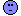See this post.

Dif-tor heh smusma 🖖
Helmut SchützThe quality of responses received is directly proportional to the quality of the question asked. 🚮
Science Quotes
d_labes
★★★

Berlin, Germany,
2017-02-06 15:06
(1449 d 14:27 ago)

@ ElMaestro
Posting: # 17023
Views: 21,152

## Kudos to ElMaestro!

Dear ElMaestro,

» ...
» Instead, I propose something along the lines of:
» pwrDK222BE=function(alpha, CV, theta0, theta1, theta2, n)
» ...

If that is really correct (what I can't verify due to a walnut-sized brain) it deserves also a barnstar!

Regards,

Detlew
Helmut
★★★Vienna, Austria,
2017-02-06 17:16
(1449 d 12:17 ago)

@ d_labes
Posting: # 17024
Views: 21,233

## Plausible

Dear Detlew,

» If that is really correct (what I can't verify due to a walnut-sized brain) it deserves also a barnstar!

Plausible to me. When I compared zizou’s results for GMR 0.95, n 24, CV 0.1–0.3 (nsims=1E6) with our Captain’s I got:

muddle <- lm(EM ~ ZZ) summary(muddle) Call: lm(formula = EM ~ ZZ) Residuals:          Min           1Q       Median           3Q          Max -6.59137e-04 -1.53260e-04  1.32946e-05  1.70670e-04  4.07843e-04 Coefficients:                 Estimate   Std. Error    t value Pr(>|t|)    (Intercept) -0.000105163  0.000130353   -0.80676  0.42077    ZZ           0.999885384  0.000191368 5224.92810  < 2e-16 *** --- Signif. codes:  0 ‘***’ 0.001 ‘**’ 0.01 ‘*’ 0.05 ‘.’ 0.1 ‘ ’ 1 Residual standard error: 0.000211273 on 199 degrees of freedom Multiple R-squared:  0.999993,  Adjusted R-squared:  0.999993 F-statistic: 2.72999e+07 on 1 and 199 DF,  p-value: < 2.22e-16

Dif-tor heh smusma 🖖
Helmut SchützThe quality of responses received is directly proportional to the quality of the question asked. 🚮
Science Quotes
d_labes
★★★

Berlin, Germany,
2017-02-06 20:25
(1449 d 09:08 ago)

@ Helmut
Posting: # 17025
Views: 21,131

## Plausible to me too

Dear Helmut,

» Plausible to me.

Here we are two! But plausible isn't a proof of truth. And that proof is too much for my brain. Remember: I'm only a run-of-the-mill statistician aka "Applied statistician. A second class statistician who imagines that he is a first class scientist." © Stephen Senn.

Regards,

Detlew
ElMaestro
★★★

Denmark,
2017-02-06 22:30
(1449 d 07:02 ago)

@ d_labes
Posting: # 17026
Views: 21,161

## Plausible to me too

Let P0= P(GMR<U)-P(GMR<L) regardless of what U and L are, as long as they are meaningful (U>L, and not negative). This quantitiy is easuily accessible via Power.TOST. If L and U are BE acceptance limits then P0 is power in a BE trial.

We can rewrite that as
P0=P(GMR in [L,U]), regardless of what U and L are, as long as they are meaningful (U>L, and not negative)

If we define L=0.8 and U=1.25 and GMR is within these limits, then we can say that P0 falls in (is composed of) three categories:
1. Those that have GMR in [L, 1.0]
2. Those that have GMR in [1.0, U]
3. Anything that isn’t 1 or 2.

Because “3” is anything else than 1 or 2, P1+P2+P3=P0.
and because 1 and 2 are mutually exclusive (can’t fall in both categories), except the zero-prob of GMR exactly 1.0. P1 and P2 are meaningfully additive, and P1+P2 will thus be the probability of having a passing study in which 1.0 isn’t in the acceptance range when L=0.8 and U=1.25. The probability we are looking for is P3, because this is a study fulfiling P(GMR in [L,U]) but not those that have GMR in [L, 1.0] and not those that have GMR in [1.0, U], therefore P3=P0-P1-P2, so we just plug in the relevant limits in Power.TOST and Bob’s your uncle.

Pass or fail!
ElMaestro
zizou
★

Plzeň, Czech Republic,
2017-02-06 22:42
(1449 d 06:51 ago)

@ d_labes
Posting: # 17027
Views: 20,945

## Plausible to me too

Dear all.
It's exact! Power is nothing else than probability.
Simple example:

You have 1 dice and you roll once:
probability P1 of you roll 1 is 1/6,
similarly P23 is probability that you roll 2 or 3 is 2/6, and
P45 is probability that you roll 4 or 5.
Then P1+P23+P45=5/6.
I you add P6 as probability that you roll six, the sum is equal to 1.

Similarly you have probability that 90% CI is in 80-100% (without 100%) = P1,
probability that 90% CI is in 100%-125% (without 100%) = P2,
then there is option when 90% CI is in 80-125% a 100% is in the 90% CI = P3,
there is nothing more (no other option which can happen), so the sum is the Classical Power (probability that you have 90% CI in 80-125%).

Classical Power=P1+P2+P3, where P3 is pwrDK222BE = Classical Power - P1 - P2.

P.S. ElMaestro was faster than me (but because I wrote it in different wording, I am posting it too ... hope for better understanding maybe)
d_labes
★★★

Berlin, Germany,
2017-02-07 10:01
(1448 d 19:32 ago)

@ zizou
Posting: # 17028
Views: 20,766

## THX

Dear ElMaestro, dear zizou!

Thanks for educating me.

Regards,

Detlew
Helmut
★★★Vienna, Austria,
2017-02-06 13:47
(1449 d 15:46 ago)

@ zizou
Posting: # 17021
Views: 21,331

## 3D

Hi zizou,

» I know, someone can do it better (eg. in 3D like this kind of figure).

Your wish is my command. 3D-plots for GMR 0.95. Not sure whether they are more informative than yours.

Conventional BE (rest of the world)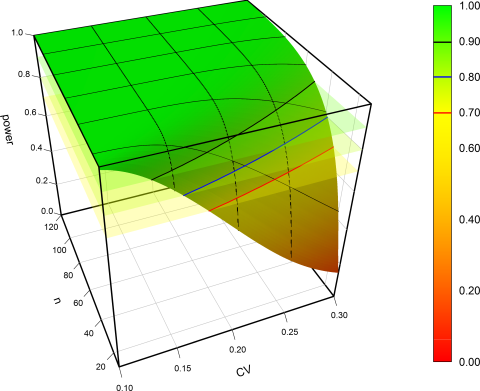Denmark: 100% included in 90% CII know only a few sample sizes by heart. One is for GMR 0.95, CV 30%, and target power 80%: 40 (expected power 81.6%). For Denmark with n=40 we get a mere 76.5%. Note the red contour lines at 70% power. As you discovered as well we never reach 80%. Fascinating the behavior at low CVs. If we increase the sample size, power decreases because a significant difference gets more likely. What a mess.

Dif-tor heh smusma 🖖
Helmut SchützThe quality of responses received is directly proportional to the quality of the question asked. 🚮
Science Quotes
d_labes
★★★

Berlin, Germany,
2017-02-06 14:54
(1449 d 14:39 ago)

@ zizou
Posting: # 17022
Views: 21,231

## Denmark Curiosa (1 in 90% CI in 0.8-1.25)

Dear zizou,

what a laborious task!One minor comment to your code:
The number of sims you used (1e4 as far as I could see) is a little bit low. Especially if you come into regions with power <=0.05.

Another comment to the Danish requirement:
Already in the early days of the evolution of (bio)equivalence tests it was dicovered that another construction of the CI may be used, namely

CI+ = (low, 1)    if high is < 1 CI+ = (1, high)   if low is >1 CI+ = (low, high) if low is <=1 and high is >=1

where low and high are the conventional confidence interval limits.
This construction of the CI always contains GMR=1 and corresponds - like the conventional CI - to a size alpha TOST.
This observation makes the Danish requirement statistical nonsense.
Unfortunately this alternative CI hasn't got further support in the history.

Berger, Hsu
"Bioequivalence Trials, Intersection-Union Tests and Equivalence Confidence Sets"
Statist. Sci. Volume 11, Number 4 (1996), 283-319.

Regards,

Detlew
zizou
★

Plzeň, Czech Republic,
2017-02-08 21:03
(1447 d 08:29 ago)

@ d_labes
Posting: # 17040
Views: 20,760

## Denmark Curiosa (1 in 90% CI in 0.8-1.25)

Dear d_labes,
thanks for comments (especially for the second).

» One minor comment to your code:
» The number of sims you used (1e4 as far as I could see) is a little bit low. Especially if you come into regions with power <=0.05.

You are right. I did the code faster for testing and unfortunately I forgot to change it to the higher number before producing figures. Nevertheless it was enough for the purpose of the post (combined with not much important subject of the post). Additionally all <=0.05 values were black in the levelplots, and I was much more interested in the power about 80 or close to 90%.

» Another comment to the Danish requirement:
» ... that another construction of the CI may be used, namely

 CI+ = (low, 1)    if high is < 1 CI+ = (1, high)   if low is >1 CI+ = (low, high) if low is <=1 and high is >=1

» where low and high are the conventional confidence interval limits.
Fascinating but nasty - it seems like a tool to make the results nicer:
• we get 95% confidence interval instead of only 90% (more accurately when true GMR=1 we get 100% CI, otherwise we get 95% CI - due to the modified construction of CIs and according to the results from Aaron Zeng R code for TOST CI simulation),
• we get always value 100% in 95% CI,
• eventually "bad" observed GMR is hidden somewhere in the 95% CI (assymetrical CI even in ln-transformed data when extended to zero), e.g. from standard 90% CI 82-86% we create little bit better looking CI 82-100% (from such CI no one knows where the observed GMR was),
• no one can calculate intra-subject variability from such CI (when lower or upper CI limit is equal to 100%) - although, it would be possible to calculate it from non-100% CI limit and observed GMR.

» This observation makes the Danish requirement statistical nonsense.
I think the observation with alternative CI is not the right reason for classifying the requirement as nonsense - alternative CI is 95% CI. EMA guideline 1401 and discussed Danish requirement, both contain the 90% confidence interval, so it is not possible to use this (same alpha 0.05, but CI widened to 95%). I had similar theoretical idea to adjust alpha, ie. to widen the CI to get the 100% in CI (valid for GMR in interval from sqrt(0.8) to 1/sqrt(0.8)). Nevertheless in the Danish requirement is stated 90% CI - no option for widening CI (even with same alpha used as in the reference).

The property of extending CI to include 100% without adjusting the alpha is interesting. I had to remind myself what the confidence interval means. I always slide in mind to probability which is common misunderstanding - citation:

"A 95% confidence interval does not mean that for a given realised interval calculated from sample data there is a 95% probability the population parameter lies within the interval. Once an experiment is done and an interval calculated, this interval either covers the parameter value or it does not; it is no longer a matter of probability. The 95% probability relates to the reliability of the estimation procedure, not to a specific calculated interval."

If it was probability (but it is not), the change of 90% CI would be to the something between 90-95% CI. For example 90% CI 87-98%, then there would be probability 5% that true GMR value is outside of the 90% CI lower and 5% outside higher. When no change of lower limit of 90% CI and adding some values up to value 100% to create a new CI, there would still be 5% probability to have true GMR lower and several percent probability to have true GMR higher than 100%. - Text in red is wrong (it's how the CI may be wrongly understood).

1) When we are constructing classical 90% CI (alpha=0.05) - the 90% of hypothetically observed confidence intervals contains the true GMR.
2) When we are constructing alternative 95% CI (alpha=0.05) - the 95% of hypothetically observed alternative confidence intervals contains the true GMR,
where alternative 95% CI is classical 90% CI extended to 100% value.

So when we use the second CI construction according to the references mentioned by d_labes (as standard 90% CI extended to have 100% in), we get 95% CI (with assumption that true GMR is not equal to 100% - it's not limiting us, in that case we get the best CI (i.e. 100% CI)).

I tried simulations (with patience) (and with reading the steps to find out what was done there), nevertheless I failed to get reported results (with seed after the # symbol).

mean(coverge.ci90) # ci90 is the classical 90% CI for difference T-R # 0.90086 mean(coverge.ci95) # ci95 is the classical 90% CI extended at one side to zero (CI is for difference T-R) # 1 # For T100=R100 it is obviously always equal to 1. Wow 100% CI! Great! Because true difference T-R is a fixed value (zero), all simulated (hypothetically observed) confidence intervals will hold the true difference.        # Otherwise (i.e. T100 differs from R100) it's about 0.95 ... due to simulations I used the word "about" but it's 0.95. (My shame I was expecting something between 0.9-0.95 until reminding myself the definitions.)
d_labes
★★★

Berlin, Germany,
2017-02-09 11:18
(1446 d 18:15 ago)

(edited by d_labes on 2017-02-09 11:43)
@ zizou
Posting: # 17041
Views: 20,593

## Alternative CI for BE decision

Dear zizou,

all you have written about CI(s) is more or less correct.
But it's a misunderstanding.

The basic test to use for the BE decision is TOST with alpha = 0.05 by convention. And this is operationally identical to the interval inclusion rule, conventionally using an ordinary 1-2*alpha CI.

The Berger, Hsu alternative CI+ is another CI (an 1-alpha CI) constructed based on TOST and gives the same result if used as decision rule for stating BE.

Code to show this via simulations:
power.sim2x2 <- function(CV, GMR, n, nsims=1E5, alpha=0.05, lBEL=0.8, uBEL=1.25,                          CItype=c("usual", "alternative"), setseed=TRUE, details=FALSE) {   ptm <- proc.time()   if(setseed) set.seed(123456)   # check CItype   CI <- match.arg(CItype)   # n is total if given as simple number   # to be correct n must then be even!   if (length(n)==1) {     nsum <- n     fact <- 2/n   }   if (length(n)==2) {     nsum <- sum(n)     fact <- 0.5*sum(1/n)   }   mse    <- log(1.0 + CV^2)   df     <- nsum-2   tval   <- qt(1-alpha,df)   # Attention! With nsims=1E8 memory of my machine (4 GB) is too low   # Thus work in chunks of 1E6 if nsims>1E6.   chunks <- 1   ns     <- nsims   if (nsims>1e6) {     chunks <- trunc(nsims/1E6)     ns     <- 1E6     if (chunks*1e6!=nsims){       nsims <- chunks*1e6       warning("nsims truncated to", nsims)     }   }   BEcount <- 0   sdm     <- sqrt(fact*mse)   mlog    <- log(GMR)   for (i in 1:chunks)   {     # simulate sample mean via its normal distribution     means  <- rnorm(ns, mean=mlog, sd=sdm)     # simulate sample mse via chi-square distribution of df*mses/mse     mses   <- mse*rchisq(ns,df)/df     hw     <- tval*sqrt(fact*mses)     lCL <- means - hw     uCL <- means + hw     if(CI=="alternative"){       # CI+, i.e. expand CI to contain GMR=1       lCL <- ifelse(lCL<0, lCL, 0)       uCL <- ifelse(uCL>0, uCL, 0)     }     # point  <- exp(means)     lCL    <- exp(lCL)     uCL    <- exp(uCL)     # standard check if CI of GMR (usual or alternative)     # is in 0.8-1.25 (without rounding CI limits)     BE     <- (lBEL<=lCL & uCL<=uBEL)     BEcount <- BEcount + sum(BE)   }   if (details) {     cat(nsims,"sims. Time elapsed (sec):\n")     print(proc.time()-ptm)   }   BEcount/nsims } 

And now try:
power.sim2x2(CV=0.2, n=24, GMR=0.95, CItype="usual")  0.89572 power.sim2x2(CV=0.2, n=24, GMR=0.95, CItype="alternative")  0.89572
Quod errat demonstrandum. At least for CV=0.2, n=24 and GMR=0.95.

BTW: It's not necessary to rely on simulations. The EMA BSWP f.i. doesn't like simulations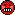. You may consider different cases for lCL, uCL to show that the alternative CI+ gives always the same decision as the usual 1-2*alpha CI.
F.i.:
If the usual CI is within the acceptance range (with upper CL below 1) -> decide BE
Then the alternative CI with upper CL set to 1 is also in the acceptance range -> decide BE.
And so on for other constellations.

Regards,

Detlew
ElMaestro
★★★

Denmark,
2017-02-09 11:36
(1446 d 17:57 ago)

@ d_labes
Posting: # 17042
Views: 20,487

## Alternative CI for BE decision

Dear Detlefffffff,

I am with zizuo here.

» all you have written about CI(s) is more or less correct.
» But it's a misunderstanding.

No, it is an understanding, and it is a case of seeing things in another and very healthy perspective.» The basic test to use for the BE decision is TOST with alpha = 0.05 by convention. And this is operationally identical to the interval inclusion rule, conventionally using an ordinary 1-2*alpha CI.

You are right, the conclusion is the same, but that is about it. The rest is to me pure and simple garbage; don't get me wrong, I am not criticising you but rather the inventor of the idea.If someone reports a CI of 0.8456-1.0000 to me, then it might in actuality imply a 90% CI of 0.8456-0.98765 or whatever. Is that science? In a sense it makes no difference as the product is approved, but hey why bother at all then with this adjustment.

Why not just go all the way and adjust all CI's so that they span across 1.0 like...what was his name... some statistician twenty-thirty years ago.... his name was Lester Hamsterballs or something...?

Now if you will excuse me, I need to leave; I have to go to the supermarket now and buy a liter of milk. The ordinary price is 0.8 Euros but I only have half a Euro. So I think I will argue that the price needs to be adjusted to my buying power, or that my 0.5 Euro coin represents a value of 0.8. I am sure the "customer service relations manager", or whatevertheheck the guy behind the till is called, will agree. Why wouldn't he?!

Pass or fail!
ElMaestro
d_labes
★★★

Berlin, Germany,
2017-02-09 11:48
(1446 d 17:45 ago)

@ ElMaestro
Posting: # 17043
Views: 20,395

## Alternative CI for BE decision

Dear ElMeastro,

to like the idea of Berger/Hsu or not is up to you.
But to like or not is not the question.

Regards,

Detlew
ElMaestro
★★★

Denmark,
2017-02-09 13:28
(1446 d 16:05 ago)

@ d_labes
Posting: # 17044
Views: 20,343

## How decidedly odd

How decidedly odd,

the guy behind the till did not allow me to leave the shop with a liter of milk against payment of 0.5 Euros?! And here I thought I had developed the perfect protocol.This was totally unexpected. I wonder what went wrong. Must talk to my psychiatrist so that I do not suffer a trauma.Pass or fail!
ElMaestro
zizou
★

Plzeň, Czech Republic,
2017-02-09 14:42
(1446 d 14:51 ago)

(edited by zizou on 2017-02-09 15:03)
@ ElMaestro
Posting: # 17046
Views: 20,540

## How decidedly odd

Dear ElMaestro,
what a pity, maybe it was not the perfect protocol. Look at this:

We could go further. Maybe you meant so, as you wrote:
» Why not just go all the way and adjust all CI's so that they span across 1.0 ...
From this reply it seems to another CI - 90% Westlake’s symmetrical confidence interval - different story.

Nevertheless what about another alternative CI (sometimes 1-alpha CI, sometimes 100% CI, and sometimes something between) which is constructed based on TOST and BE decision is not affected as well.

CI++ = (low, 1/low)     if  high < 1/low CI++ = (1/high, high)   if   low > 1/high CI++ = (low, high)      if   low = 1/high

where low and high are the classical 90% confidence interval limits.

I added the CI.9x to simulations (Aaron Zeng R code for TOST CI simulation):
set.seed(20140313) R100 <- 0.51 # Reference mean T100 <- 0.95*R100 # simulate data under alternative H, assume equal, one can change this. - Yes, I changed this. D.true <- T100 - R100 sigma <- 0.1 alpha <- 0.05 n <- 100 # sample size # simulate 100 coverage probabilities, compute means. sim.num <- 100 # generate 1000 confidence intervals for computing coverage probability rep <- 1000   coverge.ci90 <- NULL coverge.ci95 <- NULL coverge.ci9x <- NULL for(k in 1:sim.num) {  ci.90 <- NULL  ci.95 <- NULL  ci.9x <- NULL    for(i in 1:rep) {  # simulate data for Reference group  samp.R100 <- rnorm(n, mean=R100, sd=sigma)  samp.T100 <- rnorm(n, mean=T100, sd=sigma)    D.mean <- mean(samp.T100 - samp.R100)  # Assume equal variance, use pooled variance  s.pool <- sqrt((n-1)*var(samp.R100)/(2*n-2) + (n-1)*var(samp.T100)/(2*n-2))  D.se <- s.pool*sqrt(1/n + 1/n)    ci.90 <- rbind(ci.90, c(D.mean - qt(1-alpha, df = 2*n-2)*D.se,  D.mean + qt(1-alpha, df = 2*n-2)*D.se))  ci.95 <- rbind(ci.95, c(min(0, D.mean - qt(1-alpha, df = 2*n-2)*D.se),  max(0, D.mean + qt(1-alpha, df = 2*n-2)*D.se)))  ci.9x <- rbind(ci.9x, c(min(D.mean - qt(1-alpha, df = 2*n-2)*D.se, -(D.mean + qt(1-alpha, df = 2*n-2)*D.se)),  max(-(D.mean - qt(1-alpha, df = 2*n-2)*D.se), D.mean + qt(1-alpha, df = 2*n-2)*D.se)))  }  coverge.ci90 <- c(coverge.ci90, sum(1*((ci.90[, 1] <= D.true) & (ci.90[, 2] >= D.true)))/rep)  coverge.ci95 <- c(coverge.ci95, sum(1*((ci.95[, 1] <= D.true) & (ci.95[, 2] >= D.true)))/rep)  coverge.ci9x <- c(coverge.ci9x, sum(1*((ci.9x[, 1] <= D.true) & (ci.9x[, 2] >= D.true)))/rep) }   mean(coverge.ci90) # 0.90086 mean(coverge.ci95) # 0.95091 (for T100=R100 the value is 1, otherwise the value is 0.95) mean(coverge.ci9x) # 0.97507 (for different input the value seems to be from 0.95 to 1)

So when we let the 90% CI go, we can have nice (9x%) CI around 1, with alpha 0.05.
Pretty! ... Or ... Pretty nasty!

ElMaestro: You should try to buy a liter of milk for 1 Euro to verify this.Helmut
★★★Vienna, Austria,
2017-02-09 13:41
(1446 d 15:51 ago)

@ ElMaestro
Posting: # 17045
Views: 20,573

## Alternative CI for BE decision

Hi ElMaestro,

» If someone reports a CI of 0.8456-1.0000 to me, then it might in actuality imply a 90% CI of 0.8456-0.98765 or whatever. Is that science?

As zizou noted above it is less informative than the conventional (shortest) CI – we only know that the GMR is <1 – and we can’t calculate the CV from the CI any more.

» Why not just go all the way and adjust all CI's so that they span across 1.0 like...what was his name... some statistician twenty-thirty years ago.... his name was Lester Hamsterballs or something...?

41 years ago. Westlake. Wilfred J. Westlake.

Took me ages to persuade Pharsight/Certara to remove it from the standard output of WinNonlin (available till v6.3).

Dif-tor heh smusma 🖖
Helmut SchützThe quality of responses received is directly proportional to the quality of the question asked. 🚮
Science Quotes
d_labes
★★★

Berlin, Germany,
2017-02-09 20:37
(1446 d 08:56 ago)

@ Helmut
Posting: # 17047
Views: 20,419

## No alternative

Dear Helmut!

» As zizou noted above it is less informative than the conventional (shortest) CI

Beside the need to answer questions "Why does the CI not covering 1" aka "please discuss the significant treatment effect"? And answering along the line "significant treatment effect is not the question to be answered in an equivalence trial and therefore not relevant"?

» – we only know that the GMR is <1 - and we can’t calculate the CV from the CI any more.

Really? Why not?
I couldand PowerTOST also, presumed I have the point estimate at hand.

Additionally being able to re-calculate the CV from the CI is a "nice to have", but not a pre-requisite for a valid decision procedure of the (bio)equivalence.

To be clear: I don't advocate to use the alternative CI. It has it's pros and cons like the conventional 1-2*alpha CI also has it's pros and cons. See the Berger/Hsu paper and similar research. But it illustrates in my opinion that the request "GMR=1 has to be in the (whatever) CI" is superflous. It may be included in the BE devision via that alternative CI in a straight forward matter or if included in the conventional CI lead to a BE decision procedure with horrible power and ultra-conservative type I error as zizou has excellently schown and as we dicussed above. Features which renders this BE decision procedure as nearly unfeasible.

Moreover: The 1-2*alpha CI has made it, in the community of BE researchers as well as into the regulatory boddies. Thus we have no choice ... Whatever we like or like not. We are all sheeps ...

Drink milk or better drink a good Czech beer, preferrably from zizou's home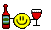.

Regards,

Detlew
ElMaestro
★★★

Denmark,
2017-02-10 13:51
(1445 d 15:42 ago)

@ d_labes
Posting: # 17048
Views: 20,170

## No alternative

Hi Detlefff and all,

» So much ado for nothing.

Wise words. I think Denmark in the grand scheme of things amounts to very little, and it isn't a market that is particularly appealing to the guys in Armani suits. This case has more academic importance, and is a good case for educational activities, but that is about it.

» Drink milk or better drink a good Czech beer, preferrably from zizou's home.

I will follow your recommendation. Thanks a lot. Will be departing for Prague this Sunday.Pass or fail!
ElMaestro
mittyri
★★

Russia,
2017-02-10 15:28
(1445 d 14:05 ago)

@ ElMaestro
Posting: # 17049
Views: 20,354

## No alternative?

Hi ElMaestro,

» Wise words. I think Denmark in the grand scheme of things amounts to very little, and it isn't a market that is particularly appealing to the guys in Armani suits.

Everything could be changed very soon:

Dr Senderovitz added: “The DMA is a committed partner of the EMA, and many of our employees are dedicated members of scientific committees and working groups under the EMA.
“There is no doubt that Brexit will have a major impact on the cooperation on medicinal products throughout Europe, especially because the UK’s Medicines and Healthcare products Regulatory Agency is currently contributing significantly to the scientific work at the EMA.
“Consequently, we are bringing more staff into our organization, and we are willing to take more than a fair share of future assessments. We are also making efforts to strengthen our capacity within scientific advice and biostatistics.”Denmark... Why not??

Kind regards,
Mittyri
d_labes
★★★

Berlin, Germany,
2017-02-10 20:10
(1445 d 09:23 ago)

@ mittyri
Posting: # 17051
Views: 20,083

## Dinamarka?

Dear mittyri,

»» Denmark... Why not??

In the light of the thread we are debating here I would opt rather for "not"!
Although ElMaestro get's his liter of milk from a supermarket compatible with the Danish twist.

I myself didn't drink milk at all, but rather a good beer.

Regards,

Detlew
d_labes
★★★

Berlin, Germany,
2017-02-10 19:57
(1445 d 09:36 ago)

@ ElMaestro
Posting: # 17050
Views: 20,078

## OT: Czech beer

Dear ElMaestro!

» » Drink milk or better drink a good Czech beer, preferrably from zizou's home.
» I will follow your recommendation. Thanks a lot. Will be departing for Prague this Sunday.

A wise decision to go to Prague! But in Prague you should enjoy Staropramen (Old spring) brewed in that city. Number #one! Really! Its my body and stomach drink every evening. Also this evening!

Regards,

Detlew
Helmut
★★★Vienna, Austria,
2019-11-08 12:47
(444 d 16:46 ago)

@ zizou
Posting: # 20765
Views: 4,052

## Denmark Curiosa (1 in 90% CI in 0.8-1.25): Gone

Hi zizou, everybody and nobody,

sorry for excavating this one.

» It was mentioned here several times that Danish Medicines Agency has one more requirement for BE:
»

"In the opinion of the Danish Medicines Agency, the 90 % confidence intervals for the ratio of the test and reference products should include 100 %, regardless that the acceptance limits are within 80.00-125.00 % or a narrower interval. Deviations are usually only accepted if it can be adequately proved that the deviation has no clinically relevant impact on the efficacy and safety of the medicinal product." source (bolded by me)

This week in Athens three assessors of the Danish agency attended the training and told that this requirement is not enforced any more. Also gone from the website updated in July 2019.

Dif-tor heh smusma 🖖
Helmut SchützThe quality of responses received is directly proportional to the quality of the question asked. 🚮
Science QuotesIng. Helmut Schütz